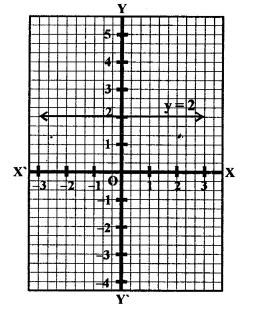## RD Sharma Class 9 Solutions Chapter 7 Introduction to Euclid’s Geometry VSAQS

These Solutions are part of RD Sharma Class 9 Solutions. Here we have given RD Sharma Class 9 Solutions Chapter 7 Introduction to Euclid’s Geometry VSAQS

Other Exercises

Question 1.
Write the equation representing x-axis.
Solution:
The equation of x-axis is, y = 0.

Question 2.
Write the equation representing y-axis.
Solution:
The equation of y-axis is, x = 0.

Question 3.
Write the equation of a line passing through the point (0, 4) and parallel to x-axis.
Solution:
The equation of the line passing through the point (0,4) and parallel to x-axis will be y = 4.

Question 4.
Write the equation of a line passing through the point (3, 5) and parallel to x-axis.
Solution:
The equation of the line passing through the point (3, 5) and parallel to x-axis will be y = 5.

Question 5.
Write the equation of a line parallel toy-axis and passing through the point (-3, -7).
Solution:
The equations of the line passing through the point (-3, -7) and parallel to y-axis will be x = -3.

Question 6.
A line passes through the point (-4, 6) and is parallel to x-axis. Find its equation. A line passes through the point (-4, 6) and is parallel to x-axis. Find its equation.
Solution:
A line parallel to x-axis and passing through the point (-4, 6) will be y = 6.

Question 7.
Solve the equation 3x – 2 = 2x + 3 and represent the solution on the number line.
Solution:
3x – 2 = 2x + 3
⇒  3x – 2x = 3 + 2 (By terms formation)
⇒  x = 5
∴ x = 5
Solution on the number line isQuestion 8.
Solve the equation 2y – 1 = y + 1 and represent it graphically on the coordinate plane.Solution:
2y – 1 = y + 1
⇒ 2y – y = 1 +1
⇒  y = 2
∴ It is a line parallel to x-axis at a distance of 2 units above the x-axis is y = 2.

Question 9.
If the point (a, 2) lies on the graph of the linear equation 2x – 3y + 8 = 0, find the value of a.
Solution:
∵
Points (a, 2) lies on the equation
2x – 3y + 8 = 0
∴ It will satisfy the equation,
Now substituting the value of x = a, y = 2 in the equation
⇒ 2a – 3 x 2+ 8= 0
⇒ 2a + 2= 0
⇒ 2a = -2
⇒ a = $$\frac { -2 }{ 2 }$$ = -1
∴ a = -1

Question 10.
Find the value of k for which the point (1, -2) lies on the graph of the linear equation, x – 2y + k = 0.
Solution:
∵ Point (1, -2) lies on the graph of the equation x – 2y + k = 0
∴ x = 1, y = -2 will satisfy the equation
Now substituting the value of x = 1, y = -2 in it
1-2 (-2) + k = 0
⇒  1 + 4 + k = 0
⇒  5+ k = 0 ⇒  k =-5
∴  k = -5

Hope given RD Sharma Class 9 Solutions Chapter 7 Introduction to Euclid’s Geometry VSAQS are helpful to complete your math homework.

If you have any doubts, please comment below. Learn Insta try to provide online math tutoring for you.